# DSA using C - Overview

## What is a Data Structure?

Data Structure is a way to organized data in such a way that it can be used efficiently. Following terms are foundation terms of a data structure.

• Interface − Each data structure has an interface. Interface represents the set of operations that a datastructure supports.An interface only provides the list of supported operations, type of parameters they can accept and return type of these operations.

• Implementation − Implementation provides the internal representation of a data structure. Implementation also provides the definition of the algorithms used in the operations of the data structure.

## Characteristics of a Data Structure

• Correctness − Data Structure implementation should implement its interface correctly.

• Time Complexity − Running time or execution time of operations of data structure must be as small as possible.

• Space Complexity − Memory usage of a data structure operation should be as little as possible.

## Need for Data Structure

As applications are getting complex and data rich, there are three common problems applications face now-a-days.

• Data Search − Consider an inventory of 1 million(106) items of a store. If application is to search an item. It has to search item in 1 million(106) items every time slowing down the search. As data grows, search will become slower.

• Processor speed − Processor speed although being very high, falls limited if data grows to billion records.

• Multiple requests − As thousands of users can search data simultaneously on a web server,even very fast server fails while searching the data.

To solve above problems, data structures come to rescue. Data can be organized in a data structure in such a way that all items may not be required to be search and required data can be searched almost instantly.

## Execution Time Cases

There are three cases which are usual used to compare various data structure's execution time in relative manner.

• Worst Case − This is the scenario where a particular data structure operation takes maximum time it can take. If a operation's worst case time is ƒ(n) then this operation will not take time more than ƒ(n) time where ƒ(n) represents function of n.

• Average Case − This is the scenario depicting the average execution time of an operation of a data structure. If a operation takes ƒ(n) time in execution then m operations will take mƒ(n) time.

• Best Case − This is the scenario depicting the least possible execution time of an operation of a data structure. If a operation takes ƒ(n) time in execution then actual operation may take time as random number which would be maximum as ƒ(n).

# DSA using C - Environment Setup

## Local Environment Setup

If you are still willing to set up your environment for C programming language, you need the following two softwares available on your computer, (a) Text Editor and (b) The C Compiler.

## Text Editor

This will be used to type your program. Examples of few editors include Windows Notepad, OS Edit command, Brief, Epsilon, EMACS, and vim or vi.

Name and version of text editor can vary on different operating systems. For example, Notepad will be used on Windows, and vim or vi can be used on windows as well as Linux or UNIX.

The files you create with your editor are called source files and contain program source code. The source files for C programs are typically named with the extension ".c".

Before starting your programming, make sure you have one text editor in place and you have enough experience to write a computer program, save it in a file, compile it and finally execute it.

## The C Compiler

The source code written in source file is the human readable source for your program. It needs to be "compiled", to turn into machine language so that your CPU can actually execute the program as per instructions given.

This C programming language compiler will be used to compile your source code into final executable program. I assume you have basic knowledge about a programming language compiler.

Most frequently used and free available compiler is GNU C/C++ compiler, otherwise you can have compilers either from HP or Solaris if you have respective Operating Systems.

Following section guides you on how to install GNU C/C++ compiler on various OS. I'm mentioning C/C++ together because GNU gcc compiler works for both C and C++ programming languages.

## Installation on UNIX/Linux

If you are using Linux or UNIX, then check whether GCC is installed on your system by entering the following command from the command line −

```\$ gcc -v
```

If you have GNU compiler installed on your machine, then it should print a message something as follows −

```Using built-in specs.
Target: i386-redhat-linux
Configured with: ../configure --prefix=/usr .......
gcc version 4.1.2 20080704 (Red Hat 4.1.2-46)
```

If GCC is not installed, then you will have to install it yourself using the detailed instructions available at https://gcc.gnu.org/install/

This tutorial has been written based on Linux and all the given examples have been compiled on Cent OS flavor of Linux system.

## Installation on Mac OS

If you use Mac OS X, the easiest way to obtain GCC is to download the Xcode development environment from Apple's web site and follow the simple installation instructions. Once you have Xcode setup, you will be able to use GNU compiler for C/C++.

Xcode is currently available at developer.apple.com/technologies/tools/.

## Installation on Windows

While installing MinWG, at a minimum, you must install gcc-core, gcc-g++, binutils, and the MinGW runtime, but you may wish to install more.

Add the bin subdirectory of your MinGW installation to your PATH environment variable, so that you can specify these tools on the command line by their simple names.

When the installation is complete, you will be able to run gcc, g++, ar, ranlib, dlltool, and several other GNU tools from the Windows command line.

# DSA using C - Algorithms

## Algorithm

Algorithm is a step by step procedure, which defines a set of instructions to be executed in certain order to get the desired output. In term of data structures, following are the categories of algorithms.

• Search − Algorithms to search an item in a datastrucure.

• Sort − Algorithms to sort items in certain order

• Insert − Algorithm to insert item in a datastructure

• Update − Algorithm to update an existing item in a data structure

• Delete − Algorithm to delete an existing item from a data structure

## Algorithm analysis

Algorithm analysis deals with the execution time or running time of various operations of a data structure. Running time of an operation can be defined as no. of computer instructions executed per operation. As exact running time of any operation varies from one computer to another computer, we usually analyze the running time of any operation as some function of n, where n is the no. of items processed in that operation in a datastructure.

## Asymptotic analysis

Asymptotic analysis refers to computing the running time of any operation in mathematical units of computation. For example, running time of one operation is computed as f(n) and of another operation as g(n2). Which means first operation running time will increase linearly with the increase in n and running time of second operation will increase exponentially when n increases. Similarly the running time of both operations will be nearly same if n is significantly small.

## Asymptotic Notations

Following are commonly used asymptotic notations used in calculating running time complexity of an algorithm.

• Ο Notation

• Ω Notation

• θ Notation

### Big Oh Notation, Ο

The Ο(n) is the formal way to express the upper bound of an algorithm's running time. It measures the worst case time complexity or longest amount of time an algorithm can possibly take to complete.

For example, for a function f(n)

Ο(f(n)) = { g(n) : there exists c > 0 and n0 such that g(n) ≤ c.f(n) for all n > n0. }

Big Oh notation is used to simplify functions. For example, we can replace a specific functional equation 7nlogn + n - 1 with Ο(f(nlogn)). Consider the scenario as follows −

7nlogn +n - 1 ≤ 7nlogn + n

7nlogn +n - 1 ≤ 7nlogn + nlogn

for n ≥ 2 so that logn ≥ 1

7nlogn +n - 1 ≤ 8nlogn

It demonstrates that f(n) = 7nlogn +n - 1 is within the range of outout of O(nlogn) using constants c = 8 and n0 = 2.

### Omega Notation, Ω

The Ω(n) is the formal way to express the lower bound of an algorithm's running time. It measures the best case time complexity or best amount of time an algorithm can possibly take to complete.

For example, for a function f(n)

Ω(f(n)) ≥ { g(n) : there exists c > 0 and n0 such that g(n) ≤ c.f(n) for all n > n0. }

### Theta Notation, θ

The θ(n) is the formal way to express both the lower bound and upper bound of an algorithm's running time. It is represented as following.

θ(f(n)) = { g(n) if and only if g(n) = Ο(f(n)) and g(n) = Ω(f(n)) for all n > n0. }

# DSA using C - Concepts

Data Structure is a way to organized data in such a way that it can be used efficiently. Following terms are basic terms of a data structure.

## Data Definition

Data Definition defines a particular data with following characteristics.

• Atomic − Definition should define a single concept

• Traceable − Definition should be be able to be mapped to some data element.

• Accurate − Definition should be unambiguous.

• Clear and Concise − Definition should be understandable.

## Data Object

Data Object represents an object having a data.

## Data Type

Data type is way to classify various types of data such as integer, string etc. which determines the values that can be used with the corresponding type of data, the type of operations that can be performed on the corresponding type of data. Data type of two types −

• Built-in Data Type

• Derived Data Type

### Built-in Data Type

Those data types for which a language has built-in support are known as Built-in Data types. For example, most of the languages provides following built-in data types.

• Integers

• Boolean (true, false)

• Floating (Decimal numbers)

• Character and Strings

### Derived Data Type

Those data types which are implementation independent as they can be implemented in one or other way are known as derived data types. These data types are normally built by combination of primary or built-in data types and associated operations on them. For example −

• List

• Array

• Stack

• Queue

# DSA using C - Arrays

## Overview

Array is a container which can hold fix number of items and these items should be of same type. Most of the datastructure make use of array to implement their algorithms. Following are important terms to understand the concepts of Array.

• Element − Each item stored in an array is called an element.

• Index − Each location of an element in an array has a numerical index which is used to identify the element.

## Array Representation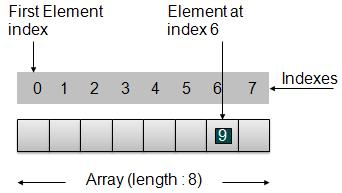As per above shown illustration, following are the important points to be considered.

• Index starts with 0.

• Array length is 8 which means it can store 8 elements.

• Each element can be accessed via its index. For example, we can fetch element at index 6 as 9.

## Basic Operations

Following are the basic operations supported by an array.

• Insertion − add an element at given index.

• Deletion − delete an element at given index.

• Search − search an element using given index or by value.

• Update − update an element at given index.

In C, when an array is initialized with size, then it assigns defaults values to its elements in following order.

Sr.No Data Type Default Value
1 bool false
2 char 0
3 int 0
4 float 0.0
5 double 0.0f
6 void
7 wchar_t 0

## Example

```#include <stdio.h>
#include <string.h>

static void display(int intArray[], int length){
int i=0;
printf("Array : [");
for(i = 0; i < length; i++) {
/* display value of element at index i. */
printf(" %d ", intArray[i]);
}
printf(" ]\n ");
}

int main() {
int i = 0;
/* Declare an array */
int intArray;

// initialize elements of array n to 0
for ( i = 0; i < 8; i++ ) {
intArray[ i ] = 0; // set elements to default value of 0;
}
printf("Array with default data.");

/* Display elements of an array.*/
display(intArray,8);

/* Operation : Insertion
Add elements in the array */
for(i = 0; i < 8; i++) {
/* place value of i at index i. */
intArray[i] = i;
}
printf("\n");
display(intArray,8);

/* Operation : Insertion
Element at any location can be updated directly */
int index = 5;
intArray[index] = 10;

printf("Array after updating element at index %d.\n",index);
display(intArray,8);

/* Operation : Search using index
Search an element using index.*/
printf("Data at index %d:%d\n" ,index,intArray[index]);

/* Operation : Search using value
Search an element using value.*/
int value = 4;
for(i = 0; i < 8; i++) {
if(intArray[i] == value ){
printf("value %d Found at index %d \n", intArray[i],i);
break;
}
}
return 0;
}
```

## Output

If we compile and run the above program then it would produce following output −

```Array with default data.Array : [ 0 0 0 0 0 0 0 0 ]

Array after adding data. Array : [ 0 1 2 3 4 5 6 7 ]
Array after updating element at index 5.
Array : [ 0 1 2 3 4 10 6 7 ]
Data at index 5: 10
4 Found at index 4
```

# DSA using C - Linked List

## Overview

Linked List is a sequence of links which contains items. Each link contains a connection to another link. Linked list the second most used data structure after array. Following are important terms to understand the concepts of Linked List.As per above shown illustration, following are the important points to be considered.

• Each Link carries a data field(s) and a Link Field called next.

• Last Link carries a Link as null to mark the end of the list.

Following are the various flavours of linked list.

• Doubly Linked List − Items can be navigated forward and backward way.

• Circular Linked List − Last item contains link of the first element as next and and first element has link to last element as prev.

## Basic Operations

Following are the basic operations supported by a list.

• Insertion − add an element at the beginning of the list.

• Deletion − delete an element at the beginning of the list.

• Display − displaying complete list.

• Search − search an element using given key.

• Delete − delete an element using given key.

## Insertion Operation

Insertion is a three step process −

• Create a new Link with provided data.```//insert link at the first location
void insertFirst(int key, int data){
struct node *link = (struct node*) malloc(sizeof(struct node));

//point it to old first node

//point first to new first node
}
```

## Deletion Operation

Deletion is a two step process −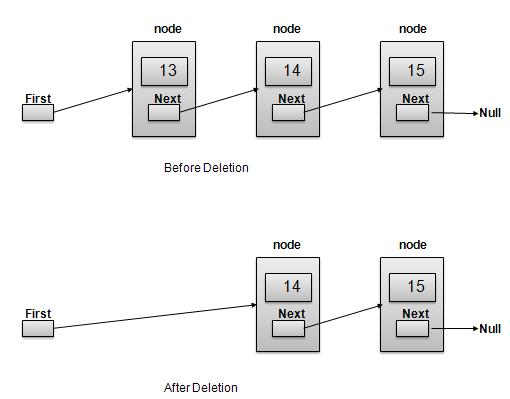```//delete first item
struct node* deleteFirst(){

//mark next to first link as first

}
```

Navigation is a recursive step process and is basis of many operations like search, delete etc. −

• Check if Current Link is not null and display it.Note −

```//display the list
void printList(){
printf("\n[ ");

//start from the beginning
while(ptr != NULL){
printf("(%d,%d) ",ptr->key,ptr->data);
ptr = ptr->next;
}
printf(" ]");
}
```

Following are the advanced operations specified for a list.

• Sort − sorting a list based on a particular order.

• Reverse − reversing a linked list.

## Sort Operation

We've used bubble sort to sort a list.

```void sort(){
int i, j, k, tempKey, tempData ;
struct node *current;
struct node *next;
int size = length();
k = size ;
for ( i = 0 ; i < size - 1 ; i++, k-- ) {
for ( j = 1 ; j < k ; j++ ) {
if ( current->data > next->data ) {
tempData = current->data ;
current->data = next->data;
next->data = tempData ;

tempKey = current->key;
current->key = next->key;
next->key = tempKey;
}
current = current->next;
next = next->next;
}
}
}
```

## Reverse Operation

Following code demonstrate reversing a single linked list.

```void reverse(struct node** head_ref) {
struct node* prev   = NULL;
struct node* next;
while (current != NULL) {
next  = current->next;
current->next = prev;
prev = current;
current = next;
}
}
```

## Example

```#include <stdio.h>
#include <string.h>
#include <stdlib.h>
#include <stdbool.h>

struct node {
int data;
int key;
struct node *next;
};
struct node *current = NULL;

//display the list
void printList(){
printf("\n[ ");

//start from the beginning
while(ptr != NULL){
printf("(%d,%d) ",ptr->key,ptr->data);
ptr = ptr->next;
}
printf(" ]");
}
//insert link at the first location
void insertFirst(int key, int data){
struct node *link = (struct node*) malloc(sizeof(struct node));

//point it to old first node

//point first to new first node
}
//delete first item
struct node* deleteFirst(){

//mark next to first link as first

}
//is list empty
bool isEmpty(){
}
int length(){
int length = 0;
struct node *current;
current = current->next){
length++;
}
return length;
}
//find a link with given key
struct node* find(int key){

//if list is empty
return NULL;
}
//navigate through list
while(current->key != key){
//if it is last node
if(current->next == NULL){
return NULL;
} else {
current = current->next;
}
}
//if data found, return the current Link
return current;
}
//delete a link with given key
struct node* delete(int key){
struct node* previous = NULL;

//if list is empty
return NULL;
}
//navigate through list
while(current->key != key){
//if it is last node
if(current->next == NULL){
return NULL;
} else {
previous = current;

current = current->next;
}
}
//found a match, update the link
//change first to point to next link
} else {
previous->next = current->next;
}
return current;
}
void sort(){
int i, j, k, tempKey, tempData ;
struct node *current;
struct node *next;
int size = length();
k = size ;
for ( i = 0 ; i < size - 1 ; i++, k-- ) {
for ( j = 1 ; j < k ; j++ ) {
if ( current->data > next->data ) {
tempData = current->data ;
current->data = next->data;
next->data = tempData ;

tempKey = current->key;
current->key = next->key;
next->key = tempKey;
}
current = current->next;
next = next->next;
}
}
}
struct node* prev   = NULL;
struct node* next;
while (current != NULL) {
next  = current->next;
current->next = prev;
prev = current;
current = next;
}
}

main() {
insertFirst(1,10);
insertFirst(2,20);
insertFirst(3,30);
insertFirst(4,1);
insertFirst(5,40);
insertFirst(6,56);

printf("Original List: ");
//print list
printList();

while(!isEmpty()){
struct node *temp = deleteFirst();
printf("\nDeleted value:");
printf("(%d,%d) ",temp->key,temp->data);
}
printf("\nList after deleting all items: ");
printList();
insertFirst(1,10);
insertFirst(2,20);
insertFirst(3,30);
insertFirst(4,1);
insertFirst(5,40);
insertFirst(6,56);
printf("\nRestored List: ");
printList();
printf("\n");

printf("Element found: ");
printf("\n");
} else {
}
delete(4);
printf("List after deleting an item: ");
printList();
printf("\n");
printf("Element found: ");
printf("\n");
} else {
}
printf("\n");
sort();
printf("List after sorting the data: ");
printList();
printf("\nList after reversing the data: ");
printList();
}
```

## Output

If we compile and run the above program then it would produce following Output −

```Original List:
[ (6,56) (5,40) (4,1) (3,30) (2,20) (1,10) ]
Deleted value:(6,56)
Deleted value:(5,40)
Deleted value:(4,1)
Deleted value:(3,30)
Deleted value:(2,20)
Deleted value:(1,10)
List after deleting all items:
[ ]
Restored List:
[ (6,56) (5,40) (4,1) (3,30) (2,20) (1,10) ]
Element found: (4,1)
List after deleting an item:
[ (6,56) (5,40) (3,30) (2,20) (1,10) ]
List after sorting the data:
[ (1,10) (2,20) (3,30) (5,40) (6,56) ]
List after reversing the data:
[ (6,56) (5,40) (3,30) (2,20) (1,10) ]
```

# DSA using C - Doubly Linked List

## Overview

Doubly Linked List is a variation of Linked list in which navigation is possible in both ways either forward and backward easily as compared to Single Linked List. Following are important terms to understand the concepts of doubly Linked List.As per above shown illustration, following are the important points to be considered.

• Each Link carries a data field(s) and a Link Field called next.

• Last Link carries a Link as null to mark the end of the list.

## Basic Operations

Following are the basic operations supported by an list.

• Insertion− add an element at the beginning of the list.

• Deletion − delete an element at the beginning of the list.

• Insert Last− add an element in the end of the list.

• Delete Last − delete an element from the end of the list.

• Insert After − add an element after an item of the list.

• Delete − delete an element from the list using key.

• Display forward − displaying complete list in forward manner.

• Display backward − displaying complete list in backward manner.

## Insertion Operation

Following code demonstrate insertion operation at beginning in a doubly linked list.

```//insert link at the first location
void insertFirst(int key, int data){
struct node *link = (struct node*) malloc(sizeof(struct node));
if(isEmpty()){
} else {
}
//point it to old first link

//point first to new first link
}
```

## Deletion Operation

Following code demonstrate deletion operation at beginning in a doubly linked list.

```//delete first item
struct node* deleteFirst(){

last = NULL;
} else {
}

}
```

## Insertion at End Operation

Following code demonstrate insertion operation at last position in a doubly linked list.

```//insert link at the last location
void insertLast(int key, int data){
struct node *link = (struct node*) malloc(sizeof(struct node));
if(isEmpty()){
} else {

//mark old last node as prev of new link
}
//point last to new last node
}
```

## Example

```#include <stdio.h>
#include <string.h>
#include <stdlib.h>
#include <stdbool.h>

struct node {
int data;
int key;
struct node *next;
struct node *prev;
};

struct node *last = NULL;
struct node *current = NULL;

//is list empty
bool isEmpty(){
}
int length(){
int length = 0;
struct node *current;
current = current->next){
length++;
}
return length;
}
//display the list in from first to last
void displayForward(){
//start from the beginning

//navigate till the end of the list
printf("\n[ ");
while(ptr != NULL){
printf("(%d,%d) ",ptr->key,ptr->data);
ptr = ptr->next;
}
printf(" ]");
}
//display the list from last to first
void displayBackward(){
//start from the last
struct node *ptr = last;

//navigate till the start of the list
printf("\n[ ");
while(ptr != NULL){
//print data
printf("(%d,%d) ",ptr->key,ptr->data);

//move to next item
ptr = ptr ->prev;
printf(" ");
}
printf(" ]");
}
//insert link at the first location
void insertFirst(int key, int data){
struct node *link = (struct node*) malloc(sizeof(struct node));
if(isEmpty()){
} else {
}
//point it to old first link

//point first to new first link
}
//insert link at the last location
void insertLast(int key, int data){
struct node *link = (struct node*) malloc(sizeof(struct node));
if(isEmpty()){
} else {

//mark old last node as prev of new link
}
//point last to new last node
}
//delete first item
struct node* deleteFirst(){

last = NULL;
} else {
}
}
//delete link at the last location
struct node* deleteLast(){

} else {
last->prev->next = NULL;
}
last = last->prev;
}
//delete a link with given key
struct node* delete(int key){
struct node* previous = NULL;

//if list is empty
return NULL;
}
//navigate through list
while(current->key != key){
//if it is last node
if(current->next == NULL){
return NULL;
} else {
previous = current;

current = current->next;
}
}
//found a match, update the link
//change first to point to next link
} else {
current->prev->next = current->next;
}
if(current == last){
//change last to point to prev link
last = current->prev;
} else {
current->next->prev = current->prev;
}
return current;
}
bool insertAfter(int key, int newKey, int data){

//if list is empty
return false;
}
//navigate through list
while(current->key != key){
//if it is last node
if(current->next == NULL){
return false;
} else {
current = current->next;
}
}
struct node *newLink = (struct node*) malloc(sizeof(struct node));

if(current==last) {
} else {
}
return true;
}
main() {
insertFirst(1,10);
insertFirst(2,20);
insertFirst(3,30);
insertFirst(4,1);
insertFirst(5,40);
insertFirst(6,56);

printf("\nList (First to Last): ");
displayForward();
printf("\n");
printf("\nList (Last to first): ");
displayBackward();

printf("\nList , after deleting first record: ");
deleteFirst();
displayForward();

printf("\nList , after deleting last record: ");
deleteLast();
displayForward();

printf("\nList , insert after key(4) : ");
insertAfter(4,7, 13);
displayForward();

printf("\nList  , after delete key(4) : ");
delete(4);
displayForward();
}
```

## Output

If we compile and run the above program then it would produce following output −

```List (First to Last):
[ (6,56) (5,40) (4,1) (3,30) (2,20) (1,10) ]

List (Last to first):
[ (1,10) (2,20) (3,30) (4,1) (5,40) (6,56) ]
List , after deleting first record:
[ (5,40) (4,1) (3,30) (2,20) (1,10) ]
List , after deleting last record:
[ (5,40) (4,1) (3,30) (2,20) ]
List , insert after key(4) :
[ (5,40) (4,1) (4,13) (3,30) (2,20) ]
List , after delete key(4) :
[ (5,40) (4,13) (3,30) (2,20) ]
```

# DSA using C - Circular Linked List

## Overview

Circular Linked List is a variation of Linked list in which first element points to last element and last element points to first element. Both Singly Linked List and Doubly Linked List can be made into as circular linked list.

## Singly Linked List as Circular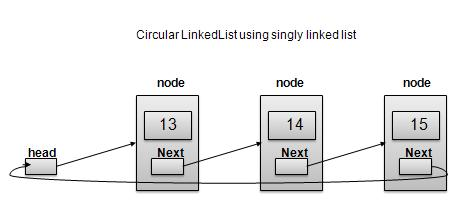## Doubly Linked List as Circular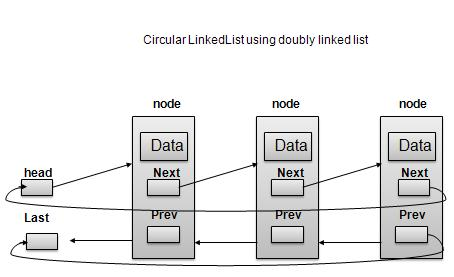As per above shown illustrations, following are the important points to be considered.

• Last Link'next points to first link of the list in both cases of singly as well as doubly linked list.

• First Link's prev points to the last of the list in case of doubly linked list.

## Basic Operations

Following are the important operations supported by a circular list.

• insert − insert an element in the start of the list.

• delete − insert an element from the start of the list.

• display − display the list.

## Length Operation

Following code demonstrate insertion operation at in a circular linked list based on single linked list.

```//insert link at the first location
void insertFirst(int key, int data){
struct node *link = (struct node*) malloc(sizeof(struct node));
if (isEmpty()) {
} else {
//point it to old first node

//point first to new first node
}
}
```

## Deletion Operation

Following code demonstrate deletion operation at in a circular linked list based on single linked list.

```//delete first item
struct node * deleteFirst(){
}
//mark next to first link as first

}
```

## Display List Operation

Following code demonstrate display list operation in a circular linked list.

```//display the list
void printList(){
printf("\n[ ");
//start from the beginning
while(ptr->next != ptr){
printf("(%d,%d) ",ptr->key,ptr->data);
ptr = ptr->next;
}
}
printf(" ]");
}
```

## Example

```#include <stdio.h>
#include <string.h>
#include <stdlib.h>
#include <stdbool.h>

struct node {
int data;
int key;
struct node *next;
};

struct node *current = NULL;

bool isEmpty(){
}
int length(){
int length = 0;

//if list is empty
return 0;
}
length++;
current = current->next;
}
return length;
}
//insert link at the first location
void insertFirst(int key, int data){
struct node *link = (struct node*) malloc(sizeof(struct node));
if (isEmpty()) {
} else {
//point it to old first node

//point first to new first node
}
}
//delete first item
struct node * deleteFirst(){
}
//mark next to first link as first

}
//display the list
void printList(){
printf("\n[ ");

//start from the beginning
while(ptr->next != ptr){
printf("(%d,%d) ",ptr->key,ptr->data);
ptr = ptr->next;
}
}
printf(" ]");
}
main() {
insertFirst(1,10);
insertFirst(2,20);
insertFirst(3,30);
insertFirst(4,1);
insertFirst(5,40);
insertFirst(6,56);
printf("Original List: ");

//print list
printList();

while(!isEmpty()){
struct node *temp = deleteFirst();
printf("\nDeleted value:");
printf("(%d,%d) ",temp->key,temp->data);
}
printf("\nList after deleting all items: ");
printList();
}
```

## Output

If we compile and run the above program then it would produce following output −

```Original List:
[ (6,56) (5,40) (4,1) (3,30) (2,20) ]
Deleted value:(6,56)
Deleted value:(5,40)
Deleted value:(4,1)
Deleted value:(3,30)
Deleted value:(2,20)
Deleted value:(1,10)
List after deleting all items:
[ ]
```

# DSA using C - Stack

## Overview

Stack is kind of data structure which allows operations on data only at one end. It allows access to the last inserted data only. Stack is also called LIFO (Last In First Out) data structure and Push and Pop operations are related in such a way that only last item pushed (added to stack) can be popped (removed from the stack).

## Stack Representation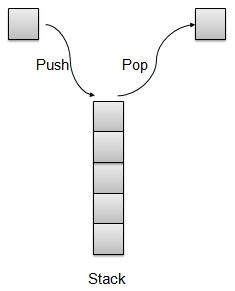## Basic Operations

Following are two primary operations of a stack which are following.

• Push − push an element at the top of the stack.

• Pop − pop an element from the top of the stack.

There is few more operations supported by stack which are following.

• Peek − get the top element of the stack.

• isFull − check if stack is full.

• isEmpty − check if stack is empty.

## Push Operation

Whenever an element is pushed into stack, stack stores that element at the top of the storage and increments the top index for later use. If storage is full then an error message is usually shown.```// Operation : Push
// push item on the top of the stack
void push(int data) {
if(!isFull()){
// increment top by 1 and insert data
intArray[++top] = data;
} else {
printf("Cannot add data. Stack is full.\n");
}
}
```

## Pop Operation

Whenever an element is to be popped from stack, stack retrives the element from the top of the storage and decrements the top index for later use.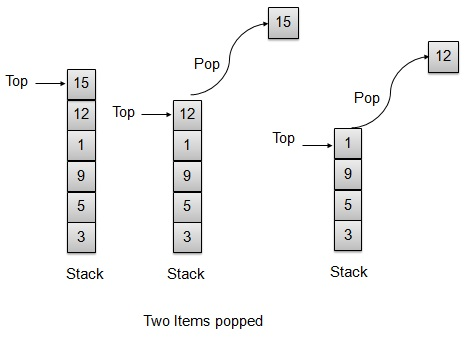```// Operation : Pop
// pop item from the top of the stack
int pop() {
//retrieve data and decrement the top by 1
return intArray[top--];
}
```

## Example

StackDemo.c

```#include <stdio.h>
#include <string.h>
#include <stdlib.h>
#include <stdbool.h>

// size of the stack
int size = 8;

// stack storage
int intArray;

// top of the stack
int top = -1;

// Operation : Pop
// pop item from the top of the stack
int pop() {
//retrieve data and decrement the top by 1
return intArray[top--];
}
// Operation : Peek
// view the data at top of the stack
int peek() {
//retrieve data from the top
return intArray[top];
}
//Operation : isFull
//return true if stack is full
bool isFull(){
return (top == size-1);
}

// Operation : isEmpty
// return true if stack is empty
bool isEmpty(){
return (top == -1);
}
// Operation : Push
// push item on the top of the stack
void push(int data) {
if(!isFull()){
// increment top by 1 and insert data
intArray[++top] = data;
} else {
printf("Cannot add data. Stack is full.\n");
}
}
main() {
// push items on to the stack
push(3);
push(5);
push(9);
push(1);
push(12);
push(15);

printf("Element at top of the stack: %d\n" ,peek());
printf("Elements: \n");

// print stack data
while(!isEmpty()){
int data = pop();
printf("%d\n",data);
}
printf("Stack full: %s\n" , isFull()?"true":"false");
printf("Stack empty: %s\n" , isEmpty()?"true":"false");
}
```

## Output

If we compile and run the above program then it would produce following output −

```Element at top of the stack: 15
Elements:
15
12
1
9
5
3
Stack full: false
Stack empty: true
```

# DSA using C - Parsing Expressions

Ordinary arithmetic expressions like 2*(3*4) are easier for human mind to parse but for an algorithm it would be pretty difficult to parse such an expression. To ease this difficulty, an arithmetic expression can be parsed by an algorithm using a two step approach.

• Transform the provided arithmetic expression to postfix notation.

• Evaluate the postfix notation.

## Infix Notation

Normal arithmetic expression follows Infix Notation in which operator is in between the operands. For example A+B here A is first operand, B is second operand and + is the operator acting on the two operands.

## Postfix Notation

Postfix notation varies from normal arithmetic expression or infix notation in a way that the operator follows the operands. For example, consider the following examples.

Sr. No. Infix Notation Postfix Notation
1 A+B AB+
2 (A+B)*C AB+C*
3 A*(B+C) ABC+*
4 A/B+C/D AB/CD/+
5 (A+B)*(C+D) AB+CD+*
6 ((A+B)*C)-D AB+C*D-

## Infix to PostFix Conversion

Before looking into the way to translate Infix to postfix notation, we need to consider following basics of infix expression evaluation.

• Evaluation of the infix expression starts from left to right.

• Keep precedence in mind, for example * has higher precedence over +. For example

• 2+3*4 = 2+12.

• 2+3*4 = 14.

• Override precedence using brackets, For example

• (2+3)*4 = 5*4.

• (2+3)*4= 20.

Now let us transform a simple infix expression A+B*C into a postfix expression manually.

Sr.No Character read Infix Expressed parsed so far Postfix expression developed so far Remarks
1 A A A
2 + A+ A
3 B A+B AB
4 * A+B* AB + can not be copied as * has higher precedence.
5 C A+B*C ABC
6 A+B*C ABC* copy * as two operands are there B and C
7 A+B*C ABC*+ copy + as two operands are there BC and A

Now let us transform the above infix expression A+B*C into a postfix expression using stack.

Sr.No Character read Infix Expressed parsed so far Postfix expression developed so far Stack Contents Remarks
1 A A A
2 + A+ A + push + operator in a stack.
3 B A+B AB +
4 * A+B* AB +* Precedence of operator * is higher than +. push * operator in the stack. Otherwise, + would pop up.
5 C A+B*C ABC +*
6 A+B*C ABC* + No more operand, pop the * operator.
7 A+B*C ABC*+ Pop the + operator.

Now let us see another example, by transforming infix expression A*(B+C) into a postfix expression using stack.

Sr.No Character read Infix Expressed parsed so far Postfix expression developed so far Stack Contents Remarks
1 A A A
2 * A* A * push * operator in a stack.
3 ( A*( A *( push ( in the stack.
4 B A*(B AB *(
5 + A*(B+ AB *(+ push + in the stack.
6 C A*(B+C ABC *(+
7 ) A*(B+C) ABC+ *( Pop the + operator.
8 A*(B+C) ABC+ * Pop the ( operator.
9 A*(B+C) ABC+* Pop the rest of the operator(s).

## Example

Now we'll demonstrate the use of stack to convert infix expression to postfix expression and then evaluate the postfix expression.

```#include<stdio.h>
#include<string.h>

//char stack
char stack;
int top=-1;

void push(char item) {
stack[++top]=item;
}
char pop() {
return stack[top--];
}

//returns precedence of operators
int precedence(char symbol) {
switch(symbol) {
case '+':
case '-':
return 2;
break;
case '*':
case '/':
return 3;
break;
case '^':
return 4;
break;
case '(':
case ')':
case '#':
return 1;
break;
}
}

//check whether the symbol is operator?
int isOperator(char symbol) {
switch(symbol){
case '+':
case '-':
case '*':
case '/':
case '^':
case '(':
case ')':
return 1;
break;
default:
return 0;
}
}
//converts infix expression to postfix
void convert(char infix[],char postfix[]){
int i,symbol,j=0;
stack[++top]='#';
for(i=0;i<strlen(infix);i++){
symbol=infix[i];
if(isOperator(symbol)==0){
postfix[j]=symbol;
j++;
} else {
if(symbol=='('){
push(symbol);
} else {
if(symbol==')'){
while(stack[top]!='('){
postfix[j]=pop();
j++;
}
pop();//pop out (.
} else {
if(precedence(symbol)>precedence(stack[top])) {
push(symbol);
} else {
while(precedence(symbol)<=precedence(stack[top])) {
postfix[j]=pop();
j++;
}
push(symbol);
}
}
}
}
}
while(stack[top]!='#') {
postfix[j]=pop();
j++;
}
postfix[j]='\0';//null terminate string.
}
//int stack
int stack_int;
int top_int=-1;

void push_int(int item) {
stack_int[++top_int]=item;
}

char pop_int() {
return stack_int[top_int--];
}
//evaluates postfix expression
int evaluate(char *postfix){
char ch;
int i=0,operand1,operand2;

while( (ch=postfix[i++]) != '\0') {
if(isdigit(ch)){
push_int(ch-'0'); // Push the operand
} else {
//Operator,pop two  operands
operand2=pop_int();
operand1=pop_int();
switch(ch) {
case '+':
push_int(operand1+operand2);
break;
case '-':
push_int(operand1-operand2);
break;
case '*':
push_int(operand1*operand2);
break;
case '/':
push_int(operand1/operand2);
break;
}
}
}
return stack_int[top_int];
}
void main() {
char infix = "1*(2+3)",postfix;
convert(infix,postfix);
printf("Infix expression is: %s\n" , infix);
printf("Postfix expression is: %s\n" , postfix);
printf("Evaluated expression is: %d\n" , evaluate(postfix));
}
```

## Output

If we compile and run the above program then it would produce following output −

```Infix expression is: 1*(2+3)
Postfix expression is: 123+*
Result is: 5
```

# DSA using C - Queue

## Overview

Queue is kind of data structure similar to stack with primary difference that the first item inserted is the first item to be removed (FIFO - First In First Out) where stack is based on LIFO, Last In First Out principal.

## Queue Representation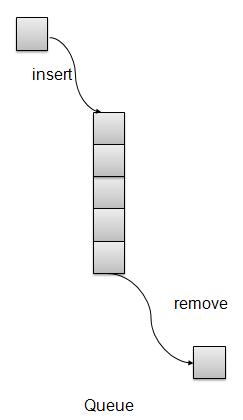## Basic Operations

• insert / enqueue − add an item to the rear of the queue.

• remove / dequeue − remove an item from the front of the queue.

We're going to implement Queue using array in this article. There is few more operations supported by queue which are following.

• Peek − get the element at front of the queue.

• isFull − check if queue is full.

• isEmpty − check if queue is empty.

## Insert / Enqueue Operation

Whenever an element is inserted into queue, queue increments the rear index for later use and stores that element at the rear end of the storage. If rear end reaches to the last index and it is wrapped to the bottom location. Such an arrangement is called wrap around and such queue is circular queue. This method is also termed as enqueue operation.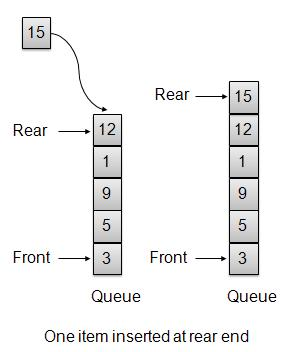```void insert(int data){
if(!isFull()){
if(rear == MAX-1){
rear = -1;
}
intArray[++rear] = data;
itemCount++;
}
}
```

## Remove / Dequeue Operation

Whenever an element is to be removed from queue, queue get the element using front index and increments the front index. As a wrap around arrangement, if front index is more than array's max index, it is set to 0.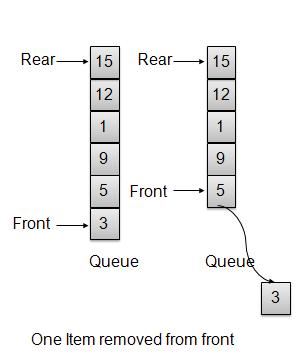```int removeData(){
int data = intArray[front++];
if(front == MAX){
front = 0;
}
itemCount--;
return data;
}
```

## Example

QueueDemo.c

```#include <stdio.h>
#include <string.h>
#include <stdlib.h>
#include <stdbool.h>
#define MAX 6

int intArray[MAX];
int front = 0;
int rear = -1;
int itemCount = 0;

int peek(){
return intArray[front];
}
bool isEmpty(){
return itemCount == 0;
}
bool isFull(){
return itemCount == MAX;
}
int size(){
return itemCount;
}
void insert(int data){
if(!isFull()){
if(rear == MAX-1){
rear = -1;
}
intArray[++rear] = data;
itemCount++;
}
}
int removeData(){
int data = intArray[front++];
if(front == MAX){
front = 0;
}
itemCount--;
return data;
}
int main() {
/* insert 5 items */
insert(3);
insert(5);
insert(9);
insert(1);
insert(12);

// front : 0
// rear  : 4
// ------------------
// index : 0 1 2 3 4
// ------------------
// queue : 3 5 9 1 12
insert(15);

// front : 0
// rear  : 5
// ---------------------
// index : 0 1 2 3 4  5
// ---------------------
// queue : 3 5 9 1 12 15
if(isFull()){
printf("Queue is full!\n");
}

// remove one item
int num = removeData();
printf("Element removed: %d\n",num);
// front : 1
// rear  : 5
// -------------------
// index : 1 2 3 4  5
// -------------------
// queue : 5 9 1 12 15

// insert more items
insert(16);

// front : 1
// rear  : -1
// ----------------------
// index : 0  1 2 3 4  5
// ----------------------
// queue : 16 5 9 1 12 15

// As queue is full, elements will not be inserted.
insert(17);
insert(18);

// ----------------------
// index : 0  1 2 3 4  5
// ----------------------
// queue : 16 5 9 1 12 15
printf("Element at front: %d\n",peek());

printf("----------------------\n");
printf("index : 5 4 3 2  1  0\n");
printf("----------------------\n");
printf("Queue:  ");
while(!isEmpty()){
int n = removeData();
printf("%d ",n);
}
}
```

## Output

If we compile and run the above program then it would produce following output −

```Queue is full!
Element removed: 3
Element at front: 5
----------------------
index : 5 4 3 2 1 0
----------------------
Queue: 5 9 1 12 15 16
```

# DSA using C - Priority Queue

## Overview

Priority Queue is more specilized data structure than Queue. Like ordinary queue, priority queue has same method but with a major difference. In Priority queue items are ordered by key value so that item with the lowest value of key is at front and item with the highest value of key is at rear or vice versa. So we're assigned priority to item based on its key value. Lower the value, higher the priority. Following are the principal methods of a Priority Queue.

## Basic Operations

• insert / enqueue − add an item to the rear of the queue.

• remove / dequeue − remove an item from the front of the queue.

## Priority Queue RepresentationWe're going to implement Queue using array in this article. There is few more operations supported by queue which are following.

• Peek − get the element at front of the queue.

• isFull − check if queue is full.

• isEmpty − check if queue is empty.

## Insert / Enqueue Operation

Whenever an element is inserted into queue, priority queue inserts the item according to its order. Here we're assuming that data with high value has low priority.```void insert(int data){
int i =0;

if(!isFull()){
// if queue is empty, insert the data
if(itemCount == 0){
intArray[itemCount++] = data;
} else {
// start from the right end of the queue
for(i = itemCount - 1; i >= 0; i-- ){
// if data is larger, shift existing item to right end
if(data > intArray[i]){
intArray[i+1] = intArray[i];
} else {
break;
}
}
// insert the data
intArray[i+1] = data;
itemCount++;
}
}
}
```

## Remove / Dequeue Operation

Whenever an element is to be removed from queue, queue get the element using item count. Once element is removed. Item count is reduced by one.```int removeData(){
return intArray[--itemCount];
}
```

## Example

```#include <stdio.h>
#include <string.h>
#include <stdlib.h>
#include <stdbool.h>
#define MAX 6

int intArray[MAX];
int itemCount = 0;

int peek(){
return intArray[itemCount - 1];
}
bool isEmpty(){
return itemCount == 0;
}
bool isFull(){
return itemCount == MAX;
}
int size(){
return itemCount;
}
void insert(int data){
int i =0;

if(!isFull()){
// if queue is empty, insert the data
if(itemCount == 0){
intArray[itemCount++] = data;
} else {
// start from the right end of the queue
for(i = itemCount - 1; i >= 0; i-- ){
// if data is larger, shift existing item to right end
if(data > intArray[i]){
intArray[i+1] = intArray[i];
} else {
break;
}
}
// insert the data
intArray[i+1] = data;
itemCount++;
}
}
}
int removeData(){
return intArray[--itemCount];
}
int main() {
/* insert 5 items */
insert(3);
insert(5);
insert(9);
insert(1);
insert(12);

// ------------------
// index : 0  1 2 3 4
// ------------------
// queue : 12 9 5 3 1
insert(15);

// ---------------------
// index : 0  1 2 3 4  5
// ---------------------
// queue : 15 12 9 5 3 1
if(isFull()){
printf("Queue is full!\n");
}

// remove one item
int num = removeData();
printf("Element removed: %d\n",num);
// ---------------------
// index : 0  1  2 3 4
// ---------------------
// queue : 15 12 9 5 3

// insert more items
insert(16);

// ----------------------
// index :  0  1 2 3 4  5
// ----------------------
// queue : 16 15 12 9 5 3

// As queue is full, elements will not be inserted.
insert(17);
insert(18);

// ----------------------
// index : 0   1  2 3 4 5
// ----------------------
// queue : 16 15 12 9 5 3
printf("Element at front: %d\n",peek());

printf("----------------------\n");
printf("index : 5 4 3 2  1  0\n");
printf("----------------------\n");
printf("Queue:  ");
while(!isEmpty()){
int n = removeData();
printf("%d ",n);
}
}
```

## Output

If we compile and run the above program then it would produce following output −

```Queue is full!
Element removed: 1
Element at front: 3
----------------------
index : 5 4 3 2 1 0
----------------------
Queue: 3 5 9 12 15 16
```

# DSA using C - Tree

## Overview

Tree represents nodes connected by edges. We'll going to discuss binary tree or binary search tree specifically.

Binary Tree is a special datastructure used for data storage purposes. A binary tree has a special condition that each node can have two children at maximum. A binary tree have benefits of both an ordered array and a linked list as search is as quick as in sorted array and insertion or deletion operation are as fast as in linked list.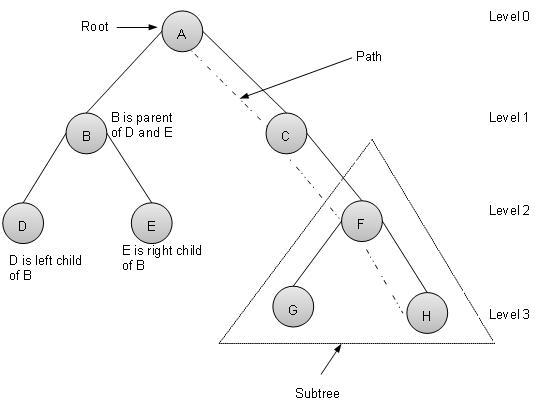## Terms

Following are important terms with respect to tree.

• Path − Path refers to sequence of nodes along the edges of a tree.

• Root − Node at the top of the tree is called root. There is only one root per tree and one path from root node to any node.

• Parent − Any node except root node has one edge upward to a node called parent.

• Child − Node below a given node connected by its edge downward is called its child node.

• Leaf − Node which does not have any child node is called leaf node.

• Subtree − Subtree represents descendents of a node.

• Visiting − Visiting refers to checking value of a node when control is on the node.

• Traversing − Traversing means passing through nodes in a specific order.

• Levels − Level of a node represents the generation of a node. If root node is at level 0, then its next child node is at level 1, its grandchild is at level 2 and so on.

• keys − Key represents a value of a node based on which a search operation is to be carried out for a node.

Binary Search tree exibits a special behaviour. A node's left child must have value less than its parent's value and node's right child must have value greater than it's parent value.

## Binary Search Tree Representation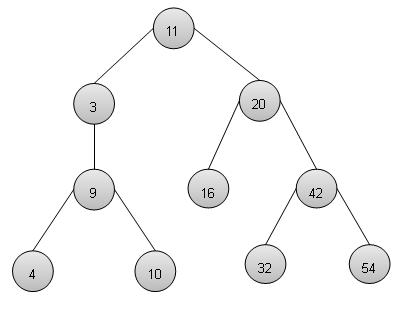We're going to implement tree using node object and connecting them through references.

## Basic Operations

Following are basic primary operations of a tree which are following.

• Search − search an element in a tree.

• Insert − insert an element in a tree.

• Preorder Traversal − traverse a tree in a preorder manner.

• Inorder Traversal − traverse a tree in an inorder manner.

• Postorder Traversal − traverse a tree in a postorder manner.

## Node

Define a node having some data, references to its left and right child nodes.

```struct node {
int data;
struct node *leftChild;
struct node *rightChild;
};
```

## Search Operation

Whenever an element is to be search. Start search from root node then if data is less than key value, search element in left subtree otherwise search element in right subtree. Follow the same algorithm for each node.

```struct node* search(int data){
struct node *current = root;
printf("Visiting elements: ");
while(current->data != data){
if(current != NULL)
printf("%d ",current->data);
//go to left tree
if(current->data > data){
current = current->leftChild;
}//else go to right tree
else{
current = current->rightChild;
}
if(current == NULL){
return NULL;
}
}
return current;
}
```

## Insert Operation

Whenever an element is to be inserted. First locate its proper location. Start search from root node then if data is less than key value, search empty location in left subtree and insert the data. Otherwise search empty location in right subtree and insert the data.

```void insert(int data){
struct node *tempNode = (struct node*) malloc(sizeof(struct node));
struct node *current;
struct node *parent;

tempNode->data = data;
tempNode->leftChild = NULL;
tempNode->rightChild = NULL;

//if tree is empty
if(root == NULL){
root = tempNode;
} else {
current = root;
parent = NULL;

while(1){
parent = current;
//go to left of the tree
if(data < parent->data){
current = current->leftChild;
//insert to the left
if(current == NULL){
parent->leftChild = tempNode;
return;
}
}//go to right of the tree
else{
current = current->rightChild;
//insert to the right
if(current == NULL){
parent->rightChild = tempNode;
return;
}
}
}
}
}
```

## Preorder Traversal

It is a simple three step process.

• visit root node

• traverse left subtree

• traverse right subtree

```void preOrder(struct node* root){
if(root!=NULL){
printf("%d ",root->data);
preOrder(root->leftChild);
preOrder(root->rightChild);
}
}
```

## Inorder Traversal

It is a simple three step process.

• traverse left subtree

• visit root node

• traverse right subtree

```void inOrder(struct node* root){
if(root!=NULL){
inOrder(root->leftChild);
printf("%d ",root->data);
inOrder(root->rightChild);
}
}
```

## Postorder Traversal

It is a simple three step process.

• traverse left subtree

• traverse right subtree

• visit root node

```void postOrder(struct node* root){
if(root!=NULL){
postOrder(root->leftChild);
postOrder(root->rightChild);
printf("%d ",root->data);
}
}
```

## Example

```#include <stdio.h>
#include <string.h>
#include <stdlib.h>
#include <stdbool.h>

struct node {
int data;
struct node *leftChild;
struct node *rightChild;
};
struct node *root = NULL;
void insert(int data){
struct node *tempNode = (struct node*) malloc(sizeof(struct node));
struct node *current;
struct node *parent;

tempNode->data = data;
tempNode->leftChild = NULL;
tempNode->rightChild = NULL;

//if tree is empty
if(root == NULL){
root = tempNode;
} else {
current = root;
parent = NULL;
while(1){
parent = current;
//go to left of the tree
if(data < parent->data){
current = current->leftChild;
//insert to the left
if(current == NULL){
parent->leftChild = tempNode;
return;
}
}//go to right of the tree
else{
current = current->rightChild;
//insert to the right
if(current == NULL){
parent->rightChild = tempNode;
return;
}
}
}
}
}
struct node* search(int data){
struct node *current = root;
printf("Visiting elements: ");
while(current->data != data){
if(current != NULL)
printf("%d ",current->data);
//go to left tree
if(current->data > data){
current = current->leftChild;
}//else go to right tree
else{
current = current->rightChild;
}
if(current == NULL){
return NULL;
}
}
return current;
}
void preOrder(struct node* root){
if(root!=NULL){
printf("%d ",root->data);
preOrder(root->leftChild);
preOrder(root->rightChild);
}
}
void inOrder(struct node* root){
if(root!=NULL){
inOrder(root->leftChild);
printf("%d ",root->data);
inOrder(root->rightChild);
}
}
void postOrder(struct node* root){
if(root!=NULL){
postOrder(root->leftChild);
postOrder(root->rightChild);
printf("%d ",root->data);
}
}
void traverse(int traversalType){
switch(traversalType){
case 1:
printf("\nPreorder traversal: ");
preOrder(root);
break;
case 2:
printf("\nInorder traversal: ");
inOrder(root);
break;
case 3:
printf("\nPostorder traversal: ");
postOrder(root);
break;
}
}

int main()
{
/*                     11               //Level 0
*/
insert(11);
/*                     11               //Level 0
*                      |
*                      |---20           //Level 1
*/
insert(20);
/*                     11               //Level 0
*                      |
*                  3---|---20           //Level 1
*/
insert(3);
/*                     11               //Level 0
*                      |
*                  3---|---20           //Level 1
*                           |
*                           |--42       //Level 2
*/
insert(42);
/*                     11               //Level 0
*                      |
*                  3---|---20           //Level 1
*                           |
*                           |--42       //Level 2
*                               |
*                               |--54   //Level 3
*/
insert(54);
/*                     11               //Level 0
*                      |
*                  3---|---20           //Level 1
*                           |
*                       16--|--42       //Level 2
*                               |
*                               |--54   //Level 3
*/
insert(16);
/*                     11               //Level 0
*                      |
*                  3---|---20           //Level 1
*                           |
*                       16--|--42       //Level 2
*                               |
*                           32--|--54   //Level 3
*/
insert(32);
/*                     11               //Level 0
*                      |
*                  3---|---20           //Level 1
*                  |        |
*                  |--9 16--|--42       //Level 2
*                               |
*                           32--|--54   //Level 3
*/
insert(9);
/*                     11               //Level 0
*                      |
*                  3---|---20           //Level 1
*                  |        |
*                  |--9 16--|--42       //Level 2
*                     |         |
*                  4--|     32--|--54   //Level 3
*/
insert(4);
/*                     11               //Level 0
*                      |
*                  3---|---20           //Level 1
*                  |        |
*                  |--9 16--|--42       //Level 2
*                     |         |
*                  4--|--10 32--|--54   //Level 3
*/
insert(10);

struct node * temp = search(32);
if(temp!=NULL){
printf("Element found.\n");
printf("( %d )",temp->data);
printf("\n");
} else {
}

struct node *node1 = search(2);
if(node1!=NULL){
printf("Element found.\n");
printf("( %d )",node1->data);
printf("\n");
} else {
}

//pre-order traversal
//root, left ,right
traverse(1);
//in-order traversal
//left, root ,right
traverse(2);
//post order traversal
//left, right, root
traverse(3);
}
```

## Output

If we compile and run the above program then it would produce following output −

```Visiting elements: 11 20 42 Element found.(32)

Preorder traversal: 11 3 9 4 10 20 16 42 32 54
Inorder traversal: 3 4 9 10 11 16 20 32 42 54
Postorder traversal: 4 10 9 3 16 32 54 42 20 11
```

# DSA using C - Hash Table

## Overview

HashTable is a datastructure in which insertion and search operations are very fast irrespective of size of the hashtable. It is nearly a constant or O(1). Hash Table uses array as a storage medium and uses hash technique to generate index where an element is to be inserted or to be located from.

## Hashing

Hashing is a technique to convert a range of key values into a range of indexes of an array. We're going to use modulo operator to get a range of key values. Consider an example of hashtable of size 20, and following items are to be stored. Item are in (key,value) format.

• (1,20)

• (2,70)

• (42,80)

• (4,25)

• (12,44)

• (14,32)

• (17,11)

• (13,78)

• (37,98)

Sr.No. Key Hash Array Index
1 1 1 % 20 = 1 1
2 2 2 % 20 = 2 2
3 42 42 % 20 = 2 2
4 4 4 % 20 = 4 4
5 12 12 % 20 = 12 12
6 14 14 % 20 = 14 14
7 17 17 % 20 = 17 17
8 13 13 % 20 = 13 13
9 37 37 % 20 = 17 17

## Linear Probing

As we can see, it may happen that the hashing technique used create already used index of the array. In such case, we can search the next empty location in the array by looking into the next cell until we found an empty cell. This technique is called linear probing.

Sr.No. Key Hash Array Index After Linear Probing, Array Index
1 1 1 % 20 = 1 1 1
2 2 2 % 20 = 2 2 2
3 42 42 % 20 = 2 2 3
4 4 4 % 20 = 4 4 4
5 12 12 % 20 = 12 12 12
6 14 14 % 20 = 14 14 14
7 17 17 % 20 = 17 17 17
8 13 13 % 20 = 13 13 13
9 37 37 % 20 = 17 17 18

## Basic Operations

Following are basic primary operations of a hashtable which are following.

• Search − search an element in a hashtable.

• Insert − insert an element in a hashtable.

• delete − delete an element from a hashtable.

## DataItem

Define a data item having some data, and key based on which search is to be conducted in hashtable.

```struct DataItem {
int data;
int key;
};
```

## Hash Method

Define a hashing method to compute the hash code of the key of the data item.

```int hashCode(int key){
return key % SIZE;
}
```

## Search Operation

Whenever an element is to be searched. Compute the hash code of the key passed and locate the element using that hashcode as index in the array. Use linear probing to get element ahead if element not found at computed hash code.

```struct DataItem *search(int key){
//get the hash
int hashIndex = hashCode(key);

//move in array until an empty
while(hashArray[hashIndex] !=NULL){
if(hashArray[hashIndex]->key == key)
return hashArray[hashIndex];
//go to next cell
++hashIndex;
//wrap around the table
hashIndex %= SIZE;
}
return NULL;
}
```

## Insert Operation

Whenever an element is to be inserted. Compute the hash code of the key passed and locate the index using that hashcode as index in the array. Use linear probing for empty location if an element is found at computed hash code.

```void insert(int key,int data){
struct DataItem *item = (struct DataItem*) malloc(sizeof(struct DataItem));
item->data = data;
item->key = key;

//get the hash
int hashIndex = hashCode(key);

//move in array until an empty or deleted cell
while(hashArray[hashIndex] !=NULL &&
hashArray[hashIndex]->key != -1){
//go to next cell
++hashIndex;
//wrap around the table
hashIndex %= SIZE;
}
hashArray[hashIndex] = item;
}
```

## Delete Operation

Whenever an element is to be deleted. Compute the hash code of the key passed and locate the index using that hashcode as index in the array. Use linear probing to get element ahead if an element is not found at computed hash code. When found, store a dummy item there to keep performance of hashtable intact.

```struct DataItem* delete(struct DataItem* item){
int key = item->key;

//get the hash
int hashIndex = hashCode(key);

//move in array until an empty
while(hashArray[hashIndex] !=NULL){
if(hashArray[hashIndex]->key == key){
struct DataItem* temp = hashArray[hashIndex];

//assign a dummy item at deleted position
hashArray[hashIndex] = dummyItem;
return temp;
}
//go to next cell
++hashIndex;
//wrap around the table
hashIndex %= SIZE;
}
return NULL;
}
```

## Example

```#include <stdio.h>
#include <string.h>
#include <stdlib.h>
#include <stdbool.h>

#define SIZE 20

struct DataItem {
int data;
int key;
};

struct DataItem* hashArray[SIZE];
struct DataItem* dummyItem;
struct DataItem* item;

int hashCode(int key){
return key % SIZE;
}

struct DataItem *search(int key){
//get the hash
int hashIndex = hashCode(key);

//move in array until an empty
while(hashArray[hashIndex] !=NULL){
if(hashArray[hashIndex]->key == key)
return hashArray[hashIndex];
//go to next cell
++hashIndex;
//wrap around the table
hashIndex %= SIZE;
}
return NULL;
}

void insert(int key,int data){
struct DataItem *item = (struct DataItem*) malloc(sizeof(struct DataItem));
item->data = data;
item->key = key;

//get the hash
int hashIndex = hashCode(key);

//move in array until an empty or deleted cell
while(hashArray[hashIndex] !=NULL &&
hashArray[hashIndex]->key != -1){
//go to next cell
++hashIndex;
//wrap around the table
hashIndex %= SIZE;
}
hashArray[hashIndex] = item;
}
struct DataItem* delete(struct DataItem* item){
int key = item->key;

//get the hash
int hashIndex = hashCode(key);

//move in array until an empty
while(hashArray[hashIndex] !=NULL){
if(hashArray[hashIndex]->key == key){
struct DataItem* temp = hashArray[hashIndex];
//assign a dummy item at deleted position
hashArray[hashIndex] = dummyItem;
return temp;
}
//go to next cell
++hashIndex;
//wrap around the table
hashIndex %= SIZE;
}
return NULL;
}
void display(){
int i=0;
for(i=0; i<SIZE; i++) {
if(hashArray[i] != NULL)
printf(" (%d,%d)",hashArray[i]->key,hashArray[i]->data);
else
printf(" ~~ ");
}
printf("\n");
}
int main(){
dummyItem = (struct DataItem*) malloc(sizeof(struct DataItem));
dummyItem->data = -1;
dummyItem->key = -1;

insert(1, 20);
insert(2, 70);
insert(42, 80);
insert(4, 25);
insert(12, 44);
insert(14, 32);
insert(17, 11);
insert(13, 78);
insert(37, 97);

display();
item = search(37);

if(item != NULL){
printf("Element found: %d\n", item->data);
} else {
}

delete(item);
item = search(37);

if(item != NULL){
printf("Element found: %d\n", item->data);
} else {
}
}
```

If we compile and run the above program then it would produce following result −

``` ~~  (1,20)  (2,70)  (42,80)  (4,25)  ~~  ~~  ~~  ~~  ~~  ~~  ~~ (12,44)  (13,78)  (14,32)  ~~  ~~  (17,11)  (37,97)  ~~
Element found: 97
```

# DSA using C - Heap

## Overview

Heap represents a special tree based data structure used to represent priority queue or for heap sort. We'll going to discuss binary heap tree specifically.

Binary heap tree can be classified as a binary tree with two constraints −

• Completeness − Binary heap tree is a complete binary tree except the last level which may not have all elements but elements from left to right should be filled in.

• Heapness − All parent nodes should be greater or smaller to their children. If parent node is to be greater than its child then it is called Max heap otherwise it is called Min heap. Max heap is used for heap sort and Min heap is used for priority queue. We're considering Min Heap and will use array implementation for the same.## Basic Operations

Following are basic primary operations of a Min heap which are following.

• Insert − insert an element in a heap.

• Get Minimum − get minimum element from the heap.

• Remove Minimum − remove the minimum element from the heap

## Insert Operation

• Whenever an element is to be inserted. Insert element at the end of the array. Increase the size of heap by 1.

• Heap up the element while heap property is broken. Compare element with parent's value and swap them if required.

```void insert(int value) {
size++;
intArray[size - 1] = value;
heapUp(size - 1);
}
void heapUp(int nodeIndex){
int parentIndex, tmp;
if (nodeIndex != 0) {
parentIndex = getParentIndex(nodeIndex);
if (intArray[parentIndex] > intArray[nodeIndex]) {
tmp = intArray[parentIndex];
intArray[parentIndex] = intArray[nodeIndex];
intArray[nodeIndex] = tmp;
heapUp(parentIndex);
}
}
}
```

## Get Minimum

Get the first element of the array implementing the heap being root.

```int getMinimum(){
return intArray;
}
```

## Remove Minimum

• Whenever an element is to be removed. Get the last element of the array and reduce size of heap by 1.

• Heap down the element while heap property is broken. Compare element with children's value and swap them if required.

```void removeMin() {
intArray = intArray[size - 1];
size--;
if (size > 0)
heapDown(0);
}
void heapDown(int nodeIndex){
int leftChildIndex, rightChildIndex, minIndex, tmp;
leftChildIndex = getLeftChildIndex(nodeIndex);
rightChildIndex = getRightChildIndex(nodeIndex);
if (rightChildIndex >= size) {
if (leftChildIndex >= size)
return;
else
minIndex = leftChildIndex;
} else {
if (intArray[leftChildIndex] <= intArray[rightChildIndex])
minIndex = leftChildIndex;
else
minIndex = rightChildIndex;
}
if (intArray[nodeIndex] > intArray[minIndex]) {
tmp = intArray[minIndex];
intArray[minIndex] = intArray[nodeIndex];
intArray[nodeIndex] = tmp;
heapDown(minIndex);
}
}
```

## Example

```#include <stdio.h>
#include <string.h>
#include <stdlib.h>
#include <stdbool.h>

int intArray;
int size;

bool isEmpty(){
return size == 0;
}
int getMinimum(){
return intArray;
}
int getLeftChildIndex(int nodeIndex){
return 2*nodeIndex +1;
}
int getRightChildIndex(int nodeIndex){
return 2*nodeIndex +2;
}
int getParentIndex(int nodeIndex){
return (nodeIndex -1)/2;
}
bool isFull(){
return size == 10;
}
/**
* Heap up the new element,until heap property is broken.
* Steps:
* 1. Compare node's value with parent's value.
* 2. Swap them, If they are in wrong order.
* */
void heapUp(int nodeIndex){
int parentIndex, tmp;
if (nodeIndex != 0) {
parentIndex = getParentIndex(nodeIndex);
if (intArray[parentIndex] > intArray[nodeIndex]) {
tmp = intArray[parentIndex];
intArray[parentIndex] = intArray[nodeIndex];
intArray[nodeIndex] = tmp;
heapUp(parentIndex);
}
}
}
/**
* Heap down the root element being least in value,until heap property is broken.
* Steps:
* 1.If current node has no children, done.
* 2.If current node has one children and heap property is broken,
* 3.Swap the current node and child node and heap down.
* 4.If current node has one children and heap property is broken, find smaller one
* 5.Swap the current node and child node and heap down.
* */
void heapDown(int nodeIndex){
int leftChildIndex, rightChildIndex, minIndex, tmp;
leftChildIndex = getLeftChildIndex(nodeIndex);
rightChildIndex = getRightChildIndex(nodeIndex);
if (rightChildIndex >= size) {
if (leftChildIndex >= size)
return;
else
minIndex = leftChildIndex;
} else {
if (intArray[leftChildIndex] <= intArray[rightChildIndex])
minIndex = leftChildIndex;
else
minIndex = rightChildIndex;
}
if (intArray[nodeIndex] > intArray[minIndex]) {
tmp = intArray[minIndex];
intArray[minIndex] = intArray[nodeIndex];
intArray[nodeIndex] = tmp;
heapDown(minIndex);
}
}
void insert(int value) {
size++;
intArray[size - 1] = value;
heapUp(size - 1);
}
void removeMin() {
intArray = intArray[size - 1];
size--;
if (size > 0)
heapDown(0);
}
main() {
/*                     5                //Level 0
*
*/
insert(5);
/*                     1                //Level 0
*                     |
*                 5---|                 //Level 1
*/
insert(1);
/*                     1                //Level 0
*                     |
*                 5---|---3             //Level 1
*/
insert(3);
/*                     1                //Level 0
*                     |
*                 5---|---3             //Level 1
*                 |
*              8--|                     //Level 2
*/
insert(8);
/*                     1                //Level 0
*                     |
*                 5---|---3             //Level 1
*                 |
*              8--|--9                  //Level 2
*/
insert(9);
/*                     1                 //Level 0
*                     |
*                 5---|---3              //Level 1
*                 |       |
*              8--|--9 6--|              //Level 2
*/
insert(6);
/*                     1                 //Level 0
*                     |
*                 5---|---2              //Level 1
*                 |       |
*              8--|--9 6--|--3           //Level 2
*/
insert(2);

printf("%d", getMinimum());

removeMin();
/*                     2                 //Level 0
*                     |
*                 5---|---3              //Level 1
*                 |       |
*              8--|--9 6--|              //Level 2
*/
printf("\n%d", getMinimum());
}
```

If we compile and run the above program then it would produce following result −

```1
2
```

# DSA using C - Graph

## Overview

Graph is a datastructure to model the mathematical graphs. It consists of a set of connected pairs called edges of vertices. We can represent a graph using an array of vertices and a two dimentional array of edges.

Important terms

• Vertex − Each node of the graph is represented as a vertex. In example given below, labeled circle represents vertices. So A to G are vertices. We can represent them using an array as shown in image below. Here A can be identified by index 0. B can be identified using index 1 and so on.

• Edge − Edge represents a path between two vertices or a line between two vertices. In example given below, lines from A to B, B to C and so on represents edges. We can use a two dimentional array to represent array as shown in image below. Here AB can be represented as 1 at row 0, column 1, BC as 1 at row 1, column 2 and so on, keeping other combinations as 0.

• Adjacency − Two node or vertices are adjacent if they are connected to each other through an edge. In example given below, B is adjacent to A, C is adjacent to B and so on.

• Path − Path represents a sequence of edges between two vertices. In example given below, ABCD represents a path from A to D.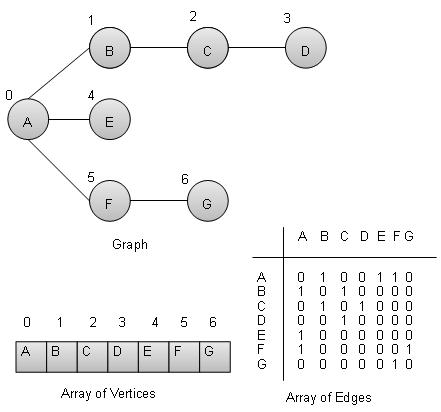## Basic Operations

Following are basic primary operations of a Graph which are following.

• Add Edge − add an edge between two vertices of a graph.

• Display Vertex − display a vertex of a graph.

```//add vertex to the vertex list
struct vertex* vertex =
(struct vertex*) malloc(sizeof(struct vertex));
vertex->label = label;
vertex->visited = false;
lstVertices[vertexCount++] = vertex;
}
```

```//add edge to edge array
}
```

## Display Edge Operation

```//display the vertex
void displayVertex(int vertexIndex){
printf("%c ",lstVertices[vertexIndex]->label);
}
```

## Traversal Algorithms

Following are important traversal algorithms on a Graph.

• Depth First Search − traverses a graph in depthwards motion.

## Depth First Search Algorithm

Depth First Search algorithm(DFS) traverses a graph in a depthward motion and uses a stack to remember to get the next vertex to start a search when a dead end occurs in any iteration.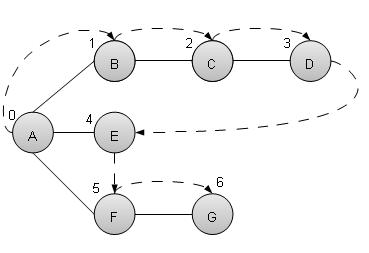As in example given above, DFS algorithm traverses from A to B to C to D first then to E, then to F and lastly to G. It employs following rules.

• Rule 1 − Visit adjacent unvisited vertex. Mark it visited. Display it. Push it in a stack.

• Rule 2 − If no adjacent vertex found, pop up a vertex from stack. (It will pop up all the vertices from the stack which do not have adjacent vertices.)

• Rule 3 − Repeat Rule 1 and Rule 2 until stack is empty.

```void depthFirstSearch(){
int i;
//mark first node as visited
lstVertices->visited = true;

//display the vertex
displayVertex(0);

//push vertex index in stack
push(0);

while(!isStackEmpty()){
//get the unvisited vertex of vertex which is at top of the stack

if(unvisitedVertex == -1){
pop();
} else {
lstVertices[unvisitedVertex]->visited = true;
displayVertex(unvisitedVertex);
push(unvisitedVertex);
}
}
//stack is empty, search is complete, reset the visited flag
for(i=0;i < vertexCount;i++){
lstVertices[i]->visited = false;
}
}
```

Breadth First Search algorithm(BFS) traverses a graph in a breadthwards motion and uses a queue to remember to get the next vertex to start a search when a dead end occurs in any iteration.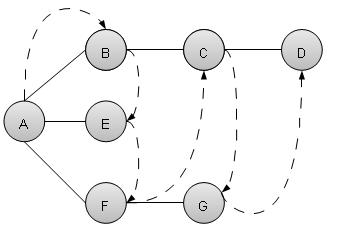As in example given above, BFS algorithm traverses from A to B to E to F first then to C and G lastly to D. It employs following rules.

• Rule 1 − Visit adjacent unvisited vertex. Mark it visited. Display it. Insert it in a queue.

• Rule 2 − If no adjacent vertex found, remove the first vertex from queue.

• Rule 3 − Repeat Rule 1 and Rule 2 until queue is empty.

```void breadthFirstSearch(){
int i;
//mark first node as visited
lstVertices->visited = true;

//display the vertex
displayVertex(0);

//insert vertex index in queue
insert(0);
int unvisitedVertex;
while(!isQueueEmpty()){
//get the unvisited vertex of vertex which is at front of the queue
int tempVertex = removeData();

lstVertices[unvisitedVertex]->visited = true;
displayVertex(unvisitedVertex);
insert(unvisitedVertex);
}
}
//queue is empty, search is complete, reset the visited flag
for(i=0;i<vertexCount;i++){
lstVertices[i]->visited = false;
}
}
```

## Example

```#include <stdio.h>
#include <string.h>
#include <stdlib.h>
#include <stdbool.h>
#define MAX 10

struct Vertex {
char label;
bool visited;
};

//stack variables
int stack[MAX];
int top=-1;

//queue variables
int queue[MAX];
int rear=-1;
int front=0;
int queueItemCount = 0;

//graph variables
//array of vertices
struct Vertex* lstVertices[MAX];

//vertex count
int vertexCount = 0;

//stack functions
void push(int item) {
stack[++top]=item;
}
int pop() {
return stack[top--];
}
int peek() {
return stack[top];
}
bool isStackEmpty(){
}
//queue functions
void insert(int data){
queue[++rear] = data;
queueItemCount++;
}
int removeData(){
queueItemCount--;
return queue[front++];
}
bool isQueueEmpty(){
return queueItemCount == 0;
}
//graph functions
//add vertex to the vertex list
struct Vertex* vertex = (struct Vertex*) malloc(sizeof(struct Vertex));
vertex->label = label;
vertex->visited = false;
lstVertices[vertexCount++] = vertex;
}
}
//display the vertex
void displayVertex(int vertexIndex){
printf("%c ",lstVertices[vertexIndex]->label);
}
int i;
for(i=0; i<vertexCount; i++)
return i;
return -1;
}
void depthFirstSearch(){
int i;
//mark first node as visited
lstVertices->visited = true;

//display the vertex
displayVertex(0);

//push vertex index in stack
push(0);

while(!isStackEmpty()){
//get the unvisited vertex of vertex which is at top of the stack

if(unvisitedVertex == -1){
pop();
} else {
lstVertices[unvisitedVertex]->visited = true;
displayVertex(unvisitedVertex);
push(unvisitedVertex);
}
}
//stack is empty, search is complete, reset the visited flag
for(i=0;i < vertexCount;i++){
lstVertices[i]->visited = false;
}
}
int i;
//mark first node as visited
lstVertices->visited = true;

//display the vertex
displayVertex(0);

//insert vertex index in queue
insert(0);
int unvisitedVertex;
while(!isQueueEmpty()){
//get the unvisited vertex of vertex which is at front of the queue
int tempVertex = removeData();

lstVertices[unvisitedVertex]->visited = true;
displayVertex(unvisitedVertex);
insert(unvisitedVertex);
}
}
//queue is empty, search is complete, reset the visited flag
for(i=0;i<vertexCount;i++){
lstVertices[i]->visited = false;
}
}
main() {
int i, j;

for(i=0; i<MAX; i++) // set adjacency
for(j=0; j<MAX; j++) // matrix to 0

/*      1  2  3
* 0  |--B--C--D
* A--|
* |
* |     4
* |-----E
* |     5  6
* |  |--F--G
* |--|
*/
printf("Depth First Search: ");

//A B C D E F G
depthFirstSearch();

//A B E F C G D
}
```

If we compile and run the above program then it would produce following result −

```Depth First Search: A B C D E F G
Breadth First Search: A B E F C G D
```

# DSA using C - Search Techniques

Search refers to locating a desired element of specified properties in a collection of items. We are going to start our discussion using following commonly used and simple search algorithms.

Sr.No Technique & Description
1

Linear Search

Linear search searches all items and its worst execution time is n where n is the number of items.

2

Binary Search

Binary search requires items to be in sorted order but its worst execution time is constant and is much faster than linear search.

3

Interpolation Search

Interpolation search requires items to be in sorted order but its worst execution time is O(n) where n is the number of items and it is much faster than linear search.

# DSA using C - Sorting Techniques

## Overview

Sorting refers to arranging data in a particular format. Sorting algorithm specifies the way to arrange data in a particular order. Most common orders are numerical or lexicographical order.

Importance of sorting lies in the fact that data searching can be optimized to a very high level if data is stored in a sorted manner. Sorting is also used to represent data in more readable formats. Some of the examples of sorting in real life scenarios are following.

• Telephone Directory − Telephone directory keeps telephone no. of people sorted on their names. So that names can be searched.

• Dictionary − Dictionary keeps words in alphabetical order so that searching of any work becomes easy.

## Types of Sorting

Following is the list of popular sorting algorithms and their comparison.

Sr.No Technique & Description
1

Bubble Sort

Bubble sort is simple to understand and implement algorithm but is very poor in performance.

2

Selection Sort

Selection sort as name specifies use the technique to select the required item and prepare sorted array accordingly.

3

Insertion Sort

Insertion sort is a variation of selection sort.

4

Shell Sort

Shell sort is an efficient version of insertion sort.

5

Quick Sort

Quick sort is a highly efficient sorting algorithm.

# DSA using C - Recursion

## Overview

Recursion refers to a technique in a programming language where a function calls itself. The function which calls itself is called a recursive method.

## Characteristics

A recursive function must posses the following two characteristics.

• Base Case(s)

• Set of rules which leads to base case after reducing the cases.

## Recursive Factorial

Factorial is one of the classical example of recursion. Factorial is a non-negative number satisfying following conditions.

• 0! = 1

• 1! = 1

• n! = n * n-1!

Factorial is represented by "!". Here Rule 1 and Rule 2 are base cases and Rule 3 are factorial rules.

As an example, 3! = 3 x 2 x 1 = 6

```int factorial(int n){
//base case
if(n == 0){
return 1;
} else {
return n * factorial(n-1);
}
}
```## Recursive Fibonacci Series

Fibonacci Series is another classical example of recursion. Fibonacci series a series of integers satisfying following conditions.

• F0 = 0

• F1 = 1

• Fn = Fn-1 + Fn-2

Fibonacci is represented by "F". Here Rule 1 and Rule 2 are base cases and Rule 3 are fibonnacci rules.

As an example, F5 = 0 1 1 2 3

## Example

```#include <stdio.h>

int factorial(int n){
//base case
if(n == 0){
return 1;
} else {
return n * factorial(n-1);
}
}
int fibbonacci(int n){
if(n ==0){
return 0;
}
else if(n==1){
return 1;
} else {
return (fibbonacci(n-1) + fibbonacci(n-2));
}
}
main(){
int n = 5;
int i;
printf("Factorial of %d: %d\n" , n , factorial(n));
printf("Fibbonacci of %d: " , n);
for(i=0;i<n;i++){
printf("%d ",fibbonacci(i));
}
}
```

## Output

If we compile and run the above program then it would produce following output −

```Factorial of 5: 120
Fibbonacci of 5: 0 1 1 2 3
```